Author: Oscar Cronquist Article last updated on October 23, 2019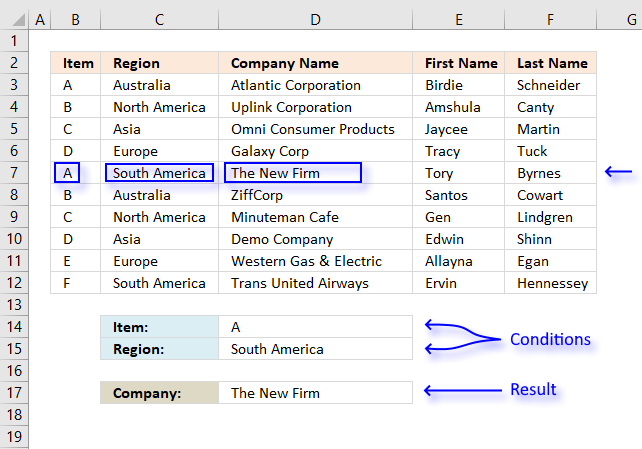I will in this article demonstrate how to use the VLOOKUP function with multiple conditions. The function was not built for these circumstances, however, I will demonstrate a few workarounds and also explain how they work.

There is a file for you to get at the very end of this article.

### How to use the VLOOKUP function with two conditions (AND logic)?The image above shows a data set in cell range B2:F12, the VLOOKUP function in cell D16 looks for both a value in column B and another value in column C. If both values match a third value on the same row is retrieved from column D and shown in cell D17.

AND logic means that the VLOOKUP function retrieves only if both conditions match.

#### Can you combine the IF function and the VLOOKUP function?

Yes, you can, in fact, it is the easiest way to VLOOKUP using two or more conditions.

Array formula in D17:

=VLOOKUP(D14, IF(C3:C12=D15, B3:F12, ""), 3, FALSE)

To enter an array formula press and hold CTRL + SHIFT simultaneously, then press Enter once. Release all keys.

The formula bar now shows the formula enclosed with curly brackets telling you that you entered the formula successfully. Don't enter the curly brackets yourself.

### Explaining formula in cell D17

I recommend you use the "Evaluate Formula" feature in Excel to examine calculations step by step.

Select cell D17, go to tab "Formulas" on the ribbon and press with left mouse button on the "Evaluate Formula" button.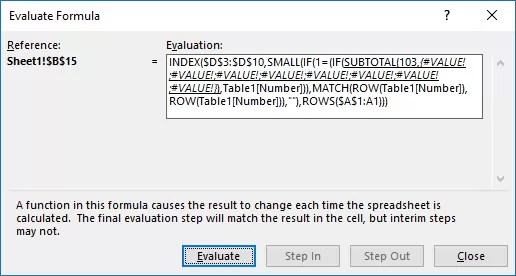(The formula shown in the above image is not the formula used in this article.)

Press with left mouse button on "Evaluate" button to see the next step in the formula calculations.

#### Step 1 - Filter records

The IF function filters records that match the value in cell D15, all remaining records are blank. The IF function has three arguments: IF(logical_test, [value_if_true], [value_if_false])

The logical_test argument is C3:C12=D15, it checks if the values in column C are equal to the condition in cell D15. TRUE is returned if it is equal and FALSE if not equal.

IF(C3:C12=D15, B3:F12, "")

becomes

IF({"Australia"; "North America"; "Asia"; "Europe"; "South America"; "Australia"; "North America"; "Asia"; "Europe"; "South America"}="South America", B3:F12, "")

becomes

IF({FALSE; FALSE; FALSE; FALSE; TRUE; FALSE; FALSE; FALSE; FALSE; TRUE}, B3:F12, "")

If a value in the array is TRUE the corresponding values in B3:F12 are returned and FALSE returns a blank row.

IF({FALSE; FALSE; FALSE; FALSE; TRUE; FALSE; FALSE; FALSE; FALSE; TRUE}, B3:F12, "")

becomes

IF({FALSE; FALSE; FALSE; FALSE; TRUE; FALSE; FALSE; FALSE; FALSE; TRUE},{"A","Australia","Atlantic Corporation","Birdie","Schneider"; "B","North America","Uplink Corporation","Amshula","Canty"; "C","Asia","Omni Consumer Products","Jaycee","Martin"; "D","Europe","Galaxy Corp","Tracy","Tuck"; "A","South America","The New Firm","Tory","Byrnes"; "B","Australia","ZiffCorp","Santos","Cowart"; "C","North America","Minuteman Cafe","Gen","Lindgren"; "D","Asia","Demo Company","Edwin","Shinn"; "E","Europe","Western Gas & Electric","Allayna","Egan"; "F","South America","Trans United Airways","Ervin","Hennessey"}, "")

and returns

{"","","","","";"","","","","";"","","","","";"","","","","";"A","South America","The New Firm","Tory","Byrnes";"","","","","";"","","","","";"","","","","";"","","","","";"F","South America","Trans United Airways","Ervin","Hennessey"}

This array has commas and semicolons as delimiting characters, the picture below shows the array in cell range B3:F12. It is now obvious that the IF function has filtered records containing ony the condition.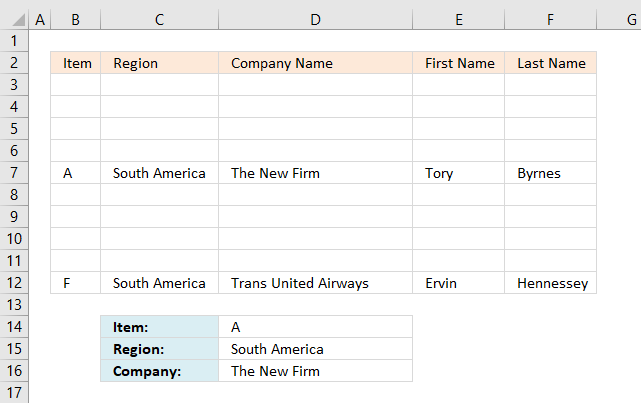#### Step 2 - VLOOKUP value and return value from column to cell D16

VLOOKUP(D14,IF(C3:C12=D15,B3:F12,""),3,FALSE)

becomes

VLOOKUP(D14, {"", "", "", "", ""; "", "", "", "", ""; "", "", "", "", ""; "", "", "", "", ""; "A", "South America", "The New Firm", "Tory", "Byrnes"; "", "", "", "", ""; "", "", "", "", ""; "", "", "", "", ""; "", "", "", "", ""; "F", "South America", "Trans United Airways", "Ervin", "Hennessey"}, 3, FALSE)

and returns "The New Firm" in cell D16.

### How to use the VLOOKUP function with two conditions applied to two columns (OR logic)?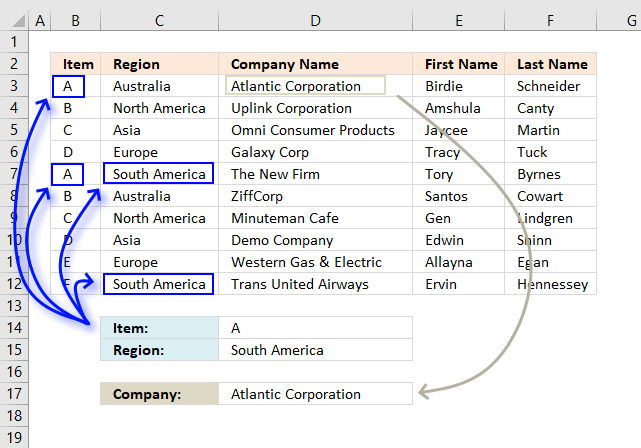This example demonstrates a formula that returns a value from a record that matches at least one of the two conditions, that is why it is called OR logic.

It becomes quite quickly obvious that the VLOOKUP function is not built for more advanced criteria, I am not using the VLOOKUP function in this example, to keep the formula as small as possible.

Array formula in cell D17:

=INDEX(\$D\$3:\$D\$12,MATCH(TRUE,(B3:B12=D14)+(C3:C12=D15)>0,0))

To enter an array formula press and hold CTRL + SHIFT simultaneously, then press Enter once. Release all keys.

The formula bar now shows the formula enclosed with curly brackets telling you that you entered the formula successfully. Don't enter the curly brackets yourself.

If you are looking for a non-array formula here is one:

=LOOKUP(2,1/((B3:B12=D14)+(C3:C12=D15)),D3:D12)

This formula returns a value from the last matching record contrary to the first formula above that returns a value from the first matching record.

### Explaining formula in cell D17

#### Step 1 - First condition

The first condition is specified in cell D14, to compare that value with the values in cell range B3:B14 I use the equal sign. It is a logical operator that returns TRUE or FALSE after the evaluations is made.

We are performing multiple calcualtions in one cell and this is the reason we need to enter this as an array formula.

B3:B12=D14

becomes

{"A";"B";"C";"D";"A";"B";"C";"D";"E";"F"}="A"

and returns

{TRUE; FALSE; FALSE; FALSE; TRUE; FALSE; FALSE; FALSE; FALSE; FALSE}

#### Step 2 - Second condition

C3:C12=D15

becomes

{"Australia";"North America";"Asia";"Europe";"South America";"Australia";"North America";"Asia";"Europe";"South America"}="South America"

and returns

{FALSE; FALSE; FALSE; FALSE; TRUE; FALSE; FALSE; FALSE; FALSE; TRUE}

#### Step 3 - Add arrays to apply OR logic

The plus sign adds the two arrays row-wise, TRUE + TRUE = 2, TRUE + FALSE = 1 and FALSE + FALSE = 0

((B3:B12=D14)+(C3:C12=D15))>0

becomes

({TRUE; FALSE; FALSE; FALSE; TRUE; FALSE; FALSE; FALSE; FALSE; FALSE} + {FALSE; FALSE; FALSE; FALSE; TRUE; FALSE; FALSE; FALSE; FALSE; TRUE})>0

becomes

{1;0;0;0;2;0;0;0;0;1}>0

and returns

{TRUE; FALSE; FALSE; FALSE; TRUE; FALSE; FALSE; FALSE; FALSE; TRUE}

#### Step 4 - Identify the position

The MATCH function, as it is set up in this example, returns the relative position of the first found matching value based on an exact match.

MATCH(TRUE,((B3:B12=D14)+(C3:C12=D15))>0,0)

becomes

MATCH(TRUE,{TRUE; FALSE; FALSE; FALSE; TRUE; FALSE; FALSE; FALSE; FALSE; TRUE},0)

and returns 1.

#### Step 5 - Return corresponding value

The INDEX function returns a value based on a row and column number.

INDEX(\$D\$3:\$D\$12, MATCH(TRUE, ((B3:B12=D14)+(C3:C12=D15))>0, 0))

becomes

INDEX(\$D\$3:\$D\$12, 1)

and returns "Atlantic Corporation" in cell D17.

### How to use the VLOOKUP function with many conditions applied to one column (OR logic)?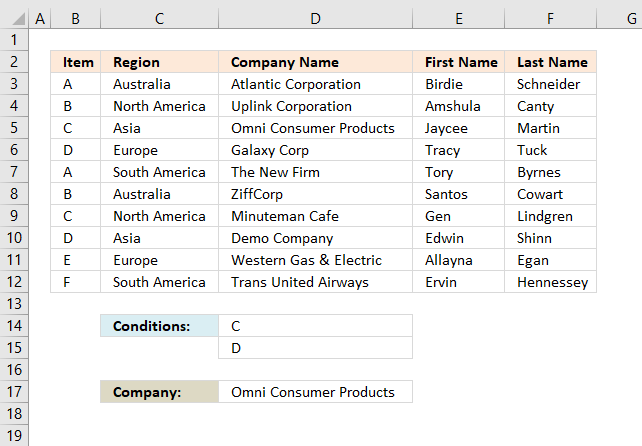This example demonstrates how to use multiple conditions and the formula returns a value from the first record that matches any of the conditions.

The following formula does not use the VLOOKUP function, it is possible to build such formula but it will be complicated and much larger than needed.

Array formula in cell D17:

=INDEX(\$D\$3:\$D\$12, MATCH(1, COUNTIF(D14:D15, B3:B12), 0))

To enter an array formula press and hold CTRL + SHIFT simultaneously, then press Enter once. Release all keys.

The formula bar now shows the formula enclosed with curly brackets telling you that you entered the formula successfully. Don't enter the curly brackets yourself.

### Explaining formula in cell D17

#### Step 1 - Check values that match

The COUNTIF function counts values that equal a condition, however, it can also count multiple conditions but we must enter this formula as an array formula in order to calculate multiple values in one cell.

COUNTIF(D14:D15, B3:B12)>0

becomes

COUNTIF({"C";"D"},{"A";"B";"C";"D";"A";"B";"C";"D";"E";"F"})

and returns

{0;0;1;1;0;0;1;1;0;0}.

This array has as many values as there are values in cell range B3:B12, the values also corresponds to B3:B12. 0 (zero) indicates that the value is not equal to "C" or "D" and 1 shows that the value is equal to "C" or "D".

#### Step 2 - Find the position of the record

The MATCH function, as it is set up in this example, returns the relative position of the first found matching value based on an exact match.

MATCH(1, COUNTIF(D14:D15, B3:B12), 0)

becomes

MATCH(1, {0;0;1;1;0;0;1;1;0;0}, 0)

and returns 3. The first value that is equal to 1 is in 3rd position in the array.

#### Step 3 - Return corresponding value

The INDEX function returns a value based on a row and column number, the cell range is in a column only, we don't need to specify the column number.

INDEX(\$D\$3:\$D\$12, MATCH(1, COUNTIF(D14:D15, B3:B12), 0))

becomes

INDEX(\$D\$3:\$D\$12, 3)

and returns "Omni Consumer Products".

### How to use VLOOKUP function with multiple lookup values?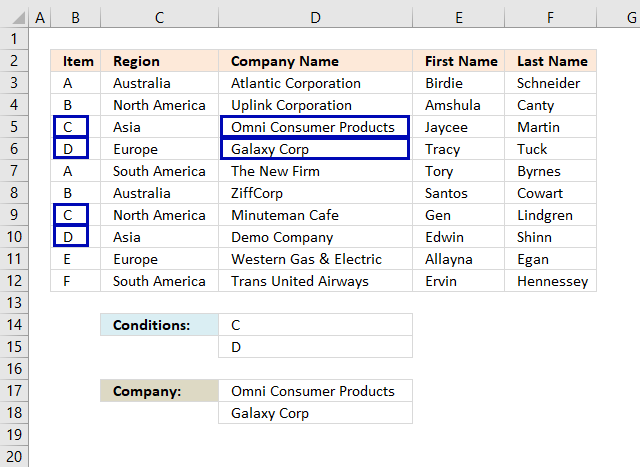This example demonstrates how to use multiple lookup values in the VLOOKUP function, the lookup values are in cell D14 and D15.

The lookup values are found in row 5,6,9 and 10 but only the corresponding values from row 5 and 6 are returned, that is how the VLOOKUP function is supposed to work. If you need to extract multiple values based on a condition read this: 5 easy ways to VLOOKUP and return multiple values

Array formula in cell D14:D15:

=VLOOKUP(D14:D15, B3:F12, 3, FALSE)

This is an array formula, it returns multiple values. We need to enter it in multiple cells at once, here is how to do it.

Select cell range D14:D15, press with left mouse button on in the formula bar to see the prompt. Copy and paste above array formula to formula bar.

To enter an array formula press and hold CTRL + SHIFT simultaneously, then press Enter once. Release all keys.

The formula bar now shows the formula enclosed with curly brackets telling you that you entered the formula successfully. Don't enter the curly brackets yourself.

The VLOOKUP function demonstrated above has two lookup values which is fine as long as you enter it in as many cells as there are lookup values.

The downside with this formula is that it only extracts one return value per lookup value, read this article: Vlookup with 2 or more lookup criteria and return multiple matches

### VLOOKUP across multiple columns?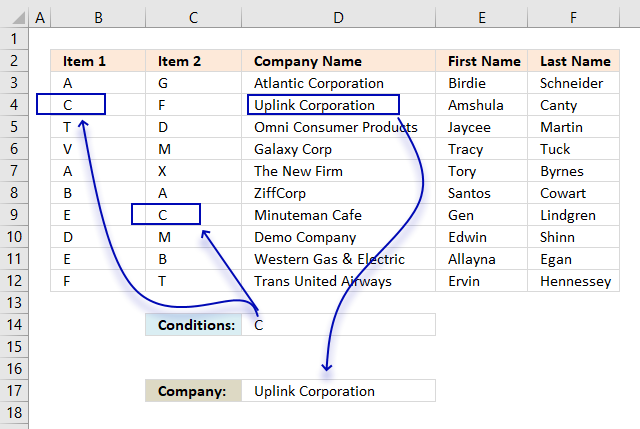Unfortunately, the VLOOKUP function can't look in other columns than the left-most column in a cell range. We need to use other functions to accomplish that.

The image above demonstrates an array formula in cell D17 that returns a value from column D (Company Name) if the corresponding value on the same row in column B or C matches the specified value in cell D17.

Array formula in cell D17:

=INDEX(\$D\$3:\$D\$12,MIN(IF(B3:C12=D14,MATCH(ROW(B3:B12),ROW(B3:B12)),"")))

To enter an array formula press and hold CTRL + SHIFT simultaneously, then press Enter once. Release all keys.

The formula bar now shows the formula enclosed with curly brackets telling you that you entered the formula successfully. Don't enter the curly brackets yourself.

### Explaining formula in cell D17

#### Step 1 - Compare values to the lookup value

The equal sign lets you check if a cell value is equal to another value, the difference with this setup is that it compares a cell value to a cell range.

In this case B3:C12, this is fine as long as you enter the formula as an array formula. The formula performs multiple calcualtions in one cell, the result is an array containing boolean values, TRUE or FALSE.

B3:C12=D14

becomes

{"A","G"; "C","F"; "T","D"; "V","M"; "A","X"; "B","A"; "E","C"; "D","M"; "E","B"; "F","T"}="C"

Values are separated by a comma or semicolon, a comma is a column delimiting character and a semicolon is a row delimiting character.

{"A","G"; "C","F"; "T","D"; "V","M"; "A","X"; "B","A"; "E","C"; "D","M"; "E","B"; "F","T"}="C"

returns

{FALSE,FALSE; TRUE,FALSE; FALSE,FALSE; FALSE,FALSE; FALSE,FALSE; FALSE,FALSE; FALSE,TRUE; FALSE,FALSE; FALSE,FALSE; FALSE,FALSE}

#### Step 2 - Replace boolean values with corresponding row number

The IF function allows you to change the array based on if the logical expression returns TRUE or FALSE.

IF(B3:C12=D14,MATCH(ROW(B3:B12),ROW(B3:B12)),"")

becomes

IF({FALSE,FALSE; TRUE,FALSE; FALSE,FALSE; FALSE,FALSE; FALSE,FALSE; FALSE,FALSE; FALSE,TRUE; FALSE,FALSE; FALSE,FALSE; FALSE,FALSE}, MATCH(ROW(B3:B12), ROW(B3:B12)),"")

becomes

IF({FALSE,FALSE; TRUE,FALSE; FALSE,FALSE; FALSE,FALSE; FALSE,FALSE; FALSE,FALSE; FALSE,TRUE; FALSE,FALSE; FALSE,FALSE; FALSE,FALSE}, {1;2;3;4;5;6;7;8;9;10}), "")

and returns

{"",""; 2,""; "",""; "",""; "",""; "",""; "",7; "",""; "",""; "",""}

#### Step 3 - Extract the smallest row number

The MIN function returns the samllest number from an cell range or array.

MIN(IF(B3:C12=D14,MATCH(ROW(B3:B12),ROW(B3:B12)),"")

becomes

MIN({"",""; 2,""; "",""; "",""; "",""; "",""; "",7; "",""; "",""; "",""})

and returns 2.

#### Step 4 - Return value

The INDEX function returns a value from a cell range or array based on a row and column number. Our example has only a single column so the column number is not needed.

INDEX(\$D\$3:\$D\$12,MIN(IF(B3:C12=D14,MATCH(ROW(B3:B12),ROW(B3:B12)),"")))

becomes

INDEX(\$D\$3:\$D\$12, 2)

### Can you concatenate VLOOKUP results?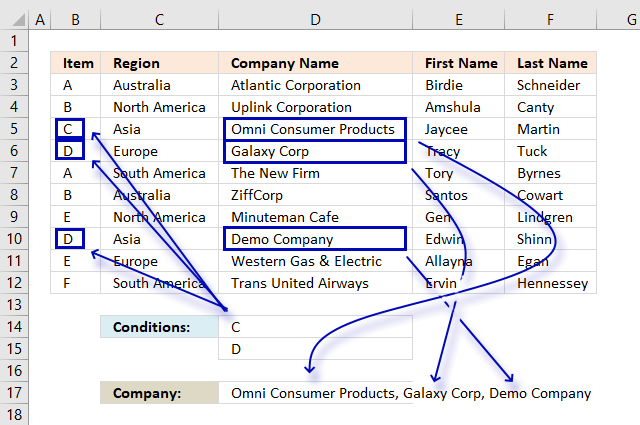No, you can't concatenate multiple return values from a VLOOKUP function. It will only return one instance. This example shows how to concatenate multiple values using multiple conditions using the TEXTJOIN, IF and COUNTIF functions.

Array formula in cell D17:

=TEXTJOIN(", ",TRUE, IF(COUNTIF(D14:D15,B3:B12),D3:D12,""))

To enter an array formula press and hold CTRL + SHIFT simultaneously, then press Enter once. Release all keys.

The formula bar now shows the formula enclosed with curly brackets telling you that you entered the formula successfully. Don't enter the curly brackets yourself.

### Explaining formula in cell D17

#### Step 1 - Determine which records match the conditions

The COUNTIF function counts values equal a condition or in this case multiple conditions.

COUNTIF(D14:D15,B3:B12)

becomes

COUNTIF({"C"; "D"} ,{"A"; "B"; "C"; "D"; "A"; "B"; "E"; "D"; "E"; "F"})

and returns {0; 0; 1; 1; 0; 0; 0; 1; 0; 0}.

#### Step 2 - Replace array with values

The IF function allows you to change the array based on if the logical expression returns TRUE or FALSE.

IF(COUNTIF(D14:D15,B3:B12),D3:D12,"")

becomes

IF({0; 0; 1; 1; 0; 0; 0; 1; 0; 0}, D3:D12, "")

becomes

IF({0; 0; 1; 1; 0; 0; 0; 1; 0; 0}, {"Atlantic Corporation"; "Uplink Corporation"; "Omni Consumer Products"; "Galaxy Corp"; "The New Firm"; "ZiffCorp"; "Minuteman Cafe"; "Demo Company"; "Western Gas & Electric"; "Trans United Airways"}, "")

and returns

{"";"";"Omni Consumer Products";"Galaxy Corp";"";"";"";"Demo Company";"";""}

#### Step 3 - Concatenate values

The TEXTJOIN function allows you to concatenate a cell range or array, you can choose the delimiting character and if you want to ignore blank values.

TEXTJOIN(", ",TRUE, IF(COUNTIF(D14:D15,B3:B12),D3:D12,""))

becomes

TEXTJOIN(", ",TRUE, {"";"";"Omni Consumer Products";"Galaxy Corp";"";"";"";"Demo Company";"";""})

and returns "Omni Consumer Products, Galaxy Corp".

### How to use VLOOKUP with dates?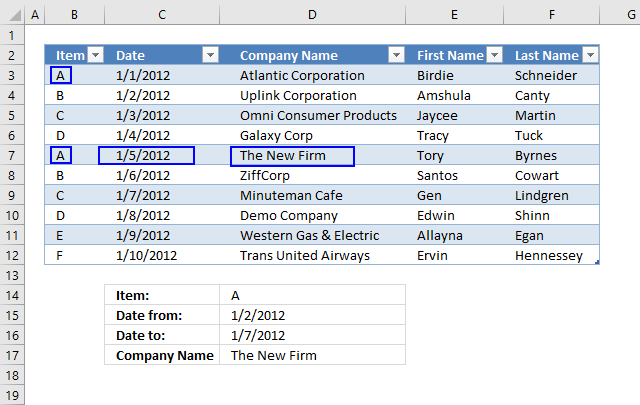This example shows how to VLOOKUP using a condition, start and end date. The formula returns a value from the first record that matches all three conditions.

Array formula in cell C5:

=VLOOKUP(C2,IF((Table3[Date]>=C3)*(Table3[Date]<=C4),Table3,""),3,FALSE)

To enter an array formula press and hold CTRL + SHIFT simultaneously, then press Enter once. Release all keys.

The formula bar now shows the formula enclosed with curly brackets telling you that you entered the formula successfully. Don't enter the curly brackets yourself.

### Explaining formula in cell C5

#### Step 1 - Identify rows with dates larger than the start date

This logical expression checks whether the dates in column Date are bigger (later) than the start date in cell C3.

Table3[Date]>=C3

becomes

{40909; 40910; 40911; 40912; 40913; 40914; 40915; 40916; 40917; 40918}>=40910

and returns

{FALSE; TRUE; TRUE; TRUE; TRUE; TRUE; TRUE; TRUE; TRUE; TRUE}

#### Step 2 - Identify rows with dates smaller than the end date

The following logical expression checks whether the dates in column Date are smaller (earlier) than the end date in cell C3.

Table3[Date]<=C4

becomes

{40909;40910;40911;40912;40913;40914;40915;40916;40917;40918}<=40915

and returns

{TRUE;TRUE;TRUE;TRUE;TRUE;TRUE;TRUE;FALSE;FALSE;FALSE}

#### Step 3 - Multiply arrays

We want to know if both conditions are met, to do that we must multiply the arrays. TRUE * TRUE = TRUE (1), TRUE * FALSE = FALSE (0), FALSE * FALSE = FALSE (0)

(Table3[Date]>=C3)*(Table3[Date]<=C4)

becomes

{FALSE; TRUE; TRUE; TRUE; TRUE; TRUE; TRUE; TRUE; TRUE; TRUE} * {TRUE; TRUE; TRUE; TRUE; TRUE; TRUE; TRUE; FALSE; FALSE; FALSE}

returns

{0;1;1;1;1;1;1;0;0;0}

#### Step 4 - Filter records that match

The IF function filters records that match both conditions.

IF((Table3[Date]>=C3)*(Table3[Date]<=C4),Table3,"")

becomes

IF({0; 1; 1; 1; 1; 1; 1; 0; 0; 0},Table3,"")

and returns

{"","","","",""; "B",40910,"Uplink Corporation","Amshula","Canty"; "C",40911,"Omni Consumer Products","Jaycee","Martin"; "D",40912,"Galaxy Corp","Tracy","Tuck"; "A",40913,"The New Firm","Tory","Byrnes"; "B",40914,"ZiffCorp","Santos","Cowart"; "C",40915,"Minuteman Cafe","Gen","Lindgren"; "","","","",""; "","","","",""; "","","","",""}.

#### Step 5 - VLOOKUP using the array

The VLOOKUP function uses the array returned from the IF function and looks for the lookup value in the left-most column in the array.

VLOOKUP(C2,IF((Table3[Date]>=C3)*(Table3[Date]<=C4),Table3,""),3,FALSE)

becomes

VLOOKUP(C2,IF({0;1;1;1;1;1;1;0;0;0},Table3,""),3,FALSE)

becomes

VLOOKUP(C2,IF({0;1;1;1;1;1;1;0;0;0},{"A", 40909, "Atlantic Corporation", "Birdie", "Schneider";"B", 40910, "Uplink Corporation", "Amshula", "Canty";"C", 40911, "Omni Consumer Products", "Jaycee", "Martin";"D", 40912, "Galaxy Corp", "Tracy", "Tuck";"A", 40913, "The New Firm", "Tory", "Byrnes";"B", 40914, "ZiffCorp", "Santos", "Cowart";"C", 40915, "Minuteman Cafe", "Gen", "Lindgren";"D", 40916, "Demo Company", "Edwin", "Shinn";"E", 40917, "Western Gas & Electric", "Allayna", "Egan";"F", 40918, "Trans United Airways", "Ervin", "Hennessey"},""),3,FALSE)

becomes

=VLOOKUP("A",{"","","","","";"B",40910,"Uplink Corporation","Amshula","Canty";"C",40911,"Omni Consumer Products","Jaycee","Martin";"D",40912,"Galaxy Corp","Tracy","Tuck";"A",40913,"The New Firm","Tory","Byrnes";"B",40914,"ZiffCorp","Santos","Cowart";"C",40915,"Minuteman Cafe","Gen","Lindgren";"","","","","";"","","","","";"","","","",""},3,FALSE)

and returns "The New Firm"

### What if I don't have the lookup column in the left-most column?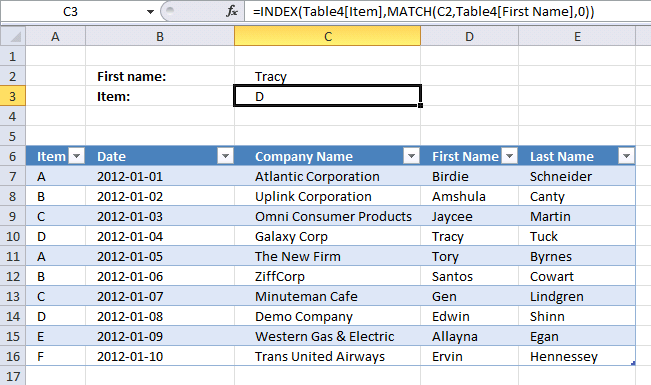The VLOOKUP function can only look for values in the first column of the table_array. The formula below demonstrates how to do a lookup in any table column and return a value from any table column.

The INDEX and MATCH function is more versatile than VLOOKUP and it is easier to apply more conditions if needed, however, it still can only return one value even if there are more records that match. The INDEX, SMALL and IF function can return multiple values, read this article: 5 easy ways to VLOOKUP and return multiple values

Formula in cell C3:

=INDEX(Table4[Item],MATCH(C2,Table4[First Name],0))

How to use the INDEX function

Gets a value in a specific cell range based on a row and column number.

How to use the MATCH function

Identify the position of a value in an array.

becomes

=INDEX(Table4[Item],4)

becomes

=INDEX({"A"; "B"; "C"; "D"; "A"; "B"; "C"; "D"; "E"; "F"},4)

and returns D in cell C3.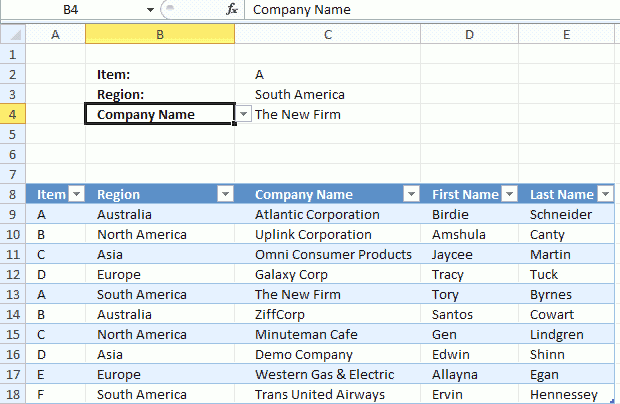The worksheet shown above lets you select the column using a drop-down list from which you want the return value, this way you don't need to edit the formula when you need data from another column.

Array formula in cell C4:

=VLOOKUP(C2, IF(Table2[Region]=C3, Table2, ""), MATCH(B4, Table2[#Headers], 0), FALSE)

#### How to create the drop down list in cell B4

1. Select cell B4
2. Go to "Data" tab
3. Press with left mouse button on "Data Validation" button
4. Select "List" i the drop down list
5. Select source: =\$C\$8:\$E\$8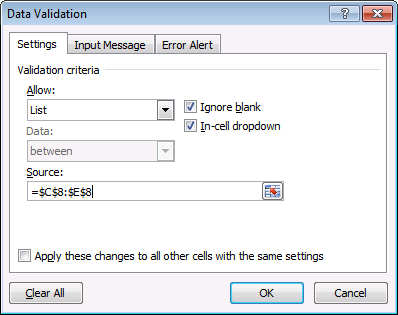6. Press with left mouse button on OK

### Explaining formula in cell C4

#### Step 1 -

VLOOKUP(C2, IF(Table2[Region]=C3, Table2, ""), MATCH(B4, Table2[#Headers], 0), FALSE)

becomes

=VLOOKUP(C2, IF(Table2[Region]=C3, Table2, ""), MATCH(Company Name", {"Item","Region","Company Name","First Name","Last Name"}, 0), FALSE)

becomes

=VLOOKUP(C2, IF(Table2[Region]=C3, Table2, ""), 3, FALSE)

and returns "The New Firm".

### Why do I want to convert the data set to an Excel Table?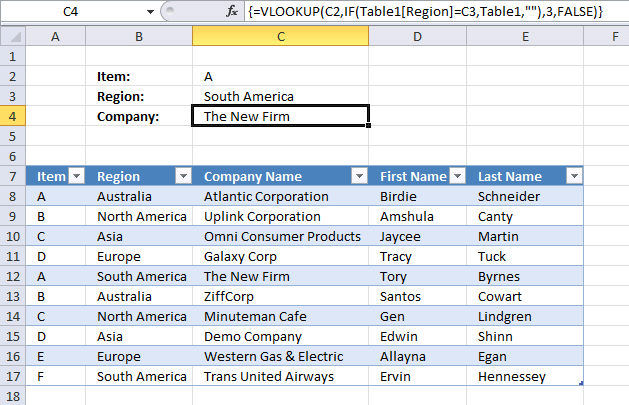If you convert your cell range to a table you can add or remove as many records to the Excel table as you want and the cell reference in the formula is automatically adjusted.

Use the table name and table column name in the VLOOKUP function to achieve this, see the formula bar in the picture above.

#### How to convert a cell range to an Excel defined Table?

1. Select any cell in your data set.
2. Go to tab "Insert" on the ribbon.
3. Press with left mouse button on "Table" button to open a dialog box.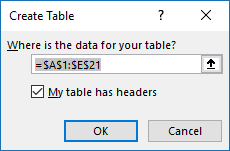4. Enable "My table has headers".
5. Press with left mouse button on OK.